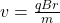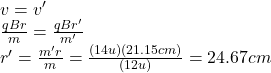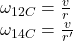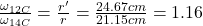## In one type of mass spectrometer, ions having the same velocity move through a uniform magnetic field. The spectrometer is being used to dis

Question

In one type of mass spectrometer, ions having the same velocity move through a uniform magnetic field. The spectrometer is being used to distinguish 12C and 14C ions. The 12C ions move in a circle of diameter 42.3 cm. Use these atomic mass values: 12C, 12.0 u; 14C, 14.0 u.

(a) What is the diameter of the orbit of 14 C+ ions?

(b) What is the ratio of the frequencies of revolution for the two types of ion?

in progress 0
5 months 2021-08-14T06:03:32+00:00 1 Answers 3 views 0

## Answers ( )

Explanation:

(a) For this problem we can use the expressionwhere B is the magnetic field, r is the radius of the curve described by the ion, m is the mass and q is the electric charge.

In this case, both isotopes have the same velocity, and we can assume that both isotopes has been ionized to have the same charge. Being r and r’ the radius of the curve for 12C ion and 14C ion respectively, we haveHence, 2*24.67cm=49.35cm is the diameter of the orbit for 14C.

(b) we can calculate ω by usingBy dividing these expressions we haveThe isotope 12C has 1.16 more angular frecuency than 14C

I hope this is useful for you

regards